David Makin's Fractal Blog

Orbits and Attractors:

More specifically this is a non-rigorous description of Orbits and Attractors for iterated formulas of the type v[n+1] = f(v[n]).

For example if the system is one dimensional and v is a real number and f(v) = 2*v then v[n+1] = 2*v[n], if the initial value of v (v) is 1 then we have:

v = 1 (= 2^0)
v = f(1) = 2*1 = 2 (= 2^1)
v = f(2) = 2*2 = 4 (= 2^2)
v = f(4) = 2*4 = 8 (= 2^3)
.
.
.
v[n] = f(2^(n-1)) = 2^n

For any iterated formula the "Orbit" is simply the sequence of values produced by the iteration, here the "Orbit" of v is the number sequence 1,2,4,8.....2^n

Clearly if this process is repeated ad nauseam then v[infinity] is infinite - hence for a starting value of v = 1 the system tends to infinity, in fact more than that - the system diverges to infinity - diverges because the magnitude of successive values of v[i] get further apart.
In fact this divergence to infinity is what happens with f(v) = 2*v for any v>0. In these cases infinity is the "Attractor" of the Orbit.

For f(v) = 2*v if v<0 then we simply get a sequence that diverges to -infinity rather than infinity so here -infinity is the attractor.

Usually if a system tends to infinity or -infinity it is just said that the system has infinity as an attractor without specifying the sign.

For f(v) = 2*v, v = 0 is a special case were all the orbit values are zero and v[infinity] = 0, here we could say that the sequence has a finite point attractor of 0.

For Fractal Art the iterated formulas used generally have more dimensions, usually 2, sometimes 3, occaissionally even more.

Normally 2 dimensional fractal formulas either use general 2 dimensional numbers (x,y) (where both x and y are real numbers) or more specifically a single complex number z where z = x + i*y (where again x and y are real numbers and i is defined as the square root of -1).

In fact for formulas more complicated than the simple example above the possible final destinations of an orbit when the formula is iterated can be classified into five distinct types:

1. The orbit may tend to infinity (usually diverging to infinity). Here infinity is the attractor.

2. It may tend to a finite point constant (usually converging to the constant i.e. such that the distance between successive orbital values decreases). Here the finite point constant is the attractor.

3. It may tend to a given sequence of finite point constants. For example a one dimensional formula may produce an orbit that tends to the sequence 1,6,4,1,6,4,1,6,4,1,6...... Here the sequence of finite point constants is the attractor, in this example the attractor consisting of the sequence of 3 values: 1,6,4 so this attractor is said to have a period of 3. This is known as a periodic attractor and the period could consist of any finite number of finite values. It should be noted that tending to the sequences 6,4,1,6,4,1... or 4,1,6,4,1,6.... would sometimes be considered as the same attractor but tending to 1,4,6,1,4,6... (or 6,1,4,6,1,4.... or 4,6,1,4,6,1....) would always be considered a different attractor.

4. It may tend to an infinite set of finite points that define a shape or shapes (a line or an area or a volume or set of lines, areas or volumes) such that the orbit never repeats but is recogniseably consistent. In many cases the shape produced by the orbit is itself a fractal. This type of attractor is usually referred to as a "Strange Attractor".

5. It may do none of the above. This could be considered as type 3 with infinite period and/or as type 4 with no defined shape or shapes.

For standard escape-time fractals you should note that a "Mandelbrot" view is a view of different functions i.e. such that f(z) in z[n+1] = f(z[n]) is different for every point in the view but the value of z is (usually) the same for all points in the view whereas in a "Julia" view the function f(z) in z[n+1) = f(z[n]) is the same for all points in the view but the value of z is different for all points in the view. Put more simply a Mandelbrot view is a view of the iterations of many related functions usually with a single starting value but a Julia view is a view of the iterations of a single function with many different starting values.

For a given f(z) different values of z (as in a Julia Set) may produce orbits that have different attractors. If a set of different values of z all produce the same attractor then that set of values of z is said to be the basin of attraction for that attractor.

Usually type 1 attraction is only considered as having one basin of attraction consisting of all z values where the attractor is infinite.
In the example Julia Set below for the complex function f(z) = z^2 - 0.06875 + 0.75625*i the coloured area consists of points where the orbits tend to infinity i.e. the coloured area shows part of the basin of attraction where the attractor is infinity (in this case all points outside the view make up the rest of this basin of attraction). Note that for all z^2+c Julias the basin of attraction for the infinity attractor is always connected.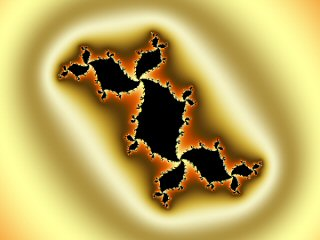The other types of attraction above can occur more than once for a given f(z), each having its own basin of attraction.

For instance a given f(z) may have two cases of type 2 attraction each with its own point attractor, here each of the point attractors would have its own basin of attraction. An example of this is the complex Newton fractal for finding the roots of z^3 - 1 = 0 in which there are 3 point attractors (the roots) each having a basin of attraction. This can be seen clearly below in the Julia mode view of the said Newton fractal where the points are coloured based on the root that the orbits converge to. The 3 basins of attraction for the point attractors are clear. Note that each of the 3 basins of attraction in this case consist of many disconnected areas - disconnected because in the infinite limit for the boundary points the result of the Newton iteration is undefined (actually these boundary points could be considered to be in the basin of attraction of a special 4th attractor but they are normally ignored).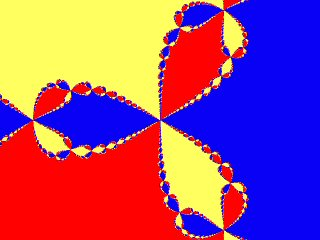As mentioned above the Mandelbrot view of an iterated formula is actually a view of the iteration of many different functions usually with the same starting value. The original Mandelbrot Set for the iteration of f(z) = z^2 + c where z and c are complex and the constant c is taken from the pixel location gives a good example of periodic attractors of different period. In this case each pixel in the Mandelbrot Set view corresponds to a point in a Julia Set with the same start and constant values. In the image of the original Mandelbrot Set below the "inside" of the Set is coloured according to the period of the attractor found for each pixel and the "outside" of the Set (where the attractor is infinity) is left black.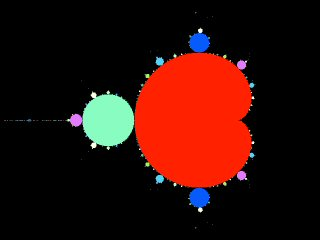Clearly the main cardioid and bulbs of the Mandelbrot Set each consist of collections of Julia Set points where the attractors have the same period. In fact the main cardioid consists of Julia Sets with single point attractors (periodic with a period of 1 i.e. type 2 attractors). The subsidiary bulbs all consist of Julia Sets which have periodic attractors (a given period for one or more given bulbs) (all with period >1 i.e. type 3 attractors).

Functions that produce types 1 to 3 attraction are generally only useful artistically speaking when viewed as Mandelbrot or Julia Sets i.e. as standard escape-time fractals where each pixel in the view has a different orbit and the pixels are coloured based on these separate orbits.

Type 4 attractors can produce artistically and/or aesthetically appealing results when simply viewing just one orbit from the attractor's basin of attraction i.e. when iterating a single function with a single start value and plotting the points from the orbit produced, colouring the pixels plotted based on various values from the single orbit or simply based on how often each pixel is "hit" by the orbit.

Below is an example image showing a type 4 attractive orbit from a variation of the "Mira" attractor, a formula that produces "Strange Attractors" for many different start values.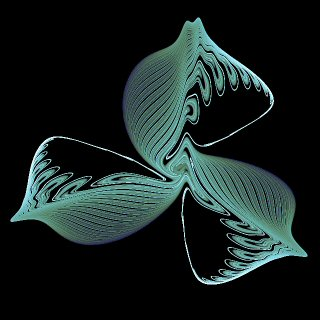The example above does seem to be a true "Strange Attractor" in that even under very high iteration counts neither divergence (to infinity) nor convergence (to a point or periodic attractor) seems to occur. Here note the "seems to" - this term is used advisedly because often it can be hard to distinguish between strange attractors and orbits that are simply very slowly divergent (to infinity), or very slowly convergent (to a point or periodic attractor), or periodic with very high periods.
As an example the image below is actually that of a convergent orbit. It very much resembles a strange attractor but when the iteration count is high enough the orbit converges to a point.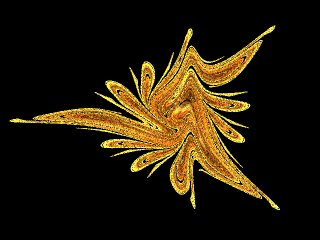Browse Galleries
Fractal Blog Archive
Art Services